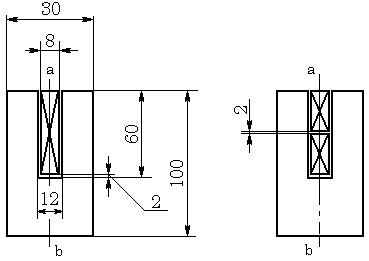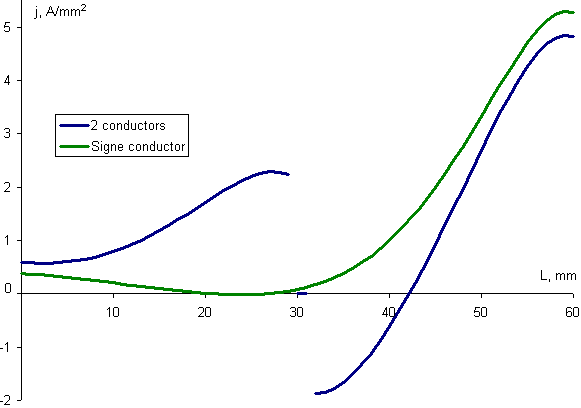QuickField

A new approach to field modelling
 Language: Global English Deutch Espanol Francais Italiano Danmark Ceske Chinese Pycckuü

Main >> Applications >> Sample problems >> Slot embedded conductor

# Exercise N 1. Slot embedded conductor

Draw a plot of current distribution within the conductor placed in the slot of electric motor.

Experiment
Current density is obtained by potential measuring along the part of conductor done by voltmeter. Phase is determined by digital phasemeter.

Problem type
Plane-parallel problem of AC magnetics.

Geometry
Problem area is to the right of symmetry axis (line ab), corresponding boundary conditions are set.Given data
Relative permeability of steel μ=100.
Relative permeability of air, copper μ= 1.
Conductivity of copper σ=57000000 S/m .
The steel is laminated, so its conductivity along the bar is σ=0 S/m.
Frequency f=50 Hz.
The total current per the bar is 600 A, or 300 A if there are two bars in the slot.

Boundary conditions
At the vertical axis of symmetry (line ab) Ht=0.
The field fades within ferromagnetics, thus at outer boundaries the field is zero A=0.

Results:
Vertical distribution of current density (phase=0) along the copper bar (L=0 at the bottom of the slot).• View simulation report in PDF
• Download simulation files (files may be viewed using any QuickField Edition)..